# NCERT Solutions for Class 6 Maths Chapter 10 – Mensuration

NCERT Solutions for Class 6 Maths Chapter 10 – Mensuration comprises of the 3 Exercises

This Chapter contains the Exercises relating to the following topics, which are discussed in Chapter 10 – Mensuration Class 6 NCERT book  : –

• 10.1 Introduction
• 10.2 Perimeter
• 10.2.1 Perimeter of a rectangle
• 10.2.2 Perimeter of regular shapes
• 10.3 Area
• 10.3.1 Area of a rectangle
• 10.3.2 Area of a square

### NCERT Solutions for Class 6 Maths Chapter 10 Exercise 10.1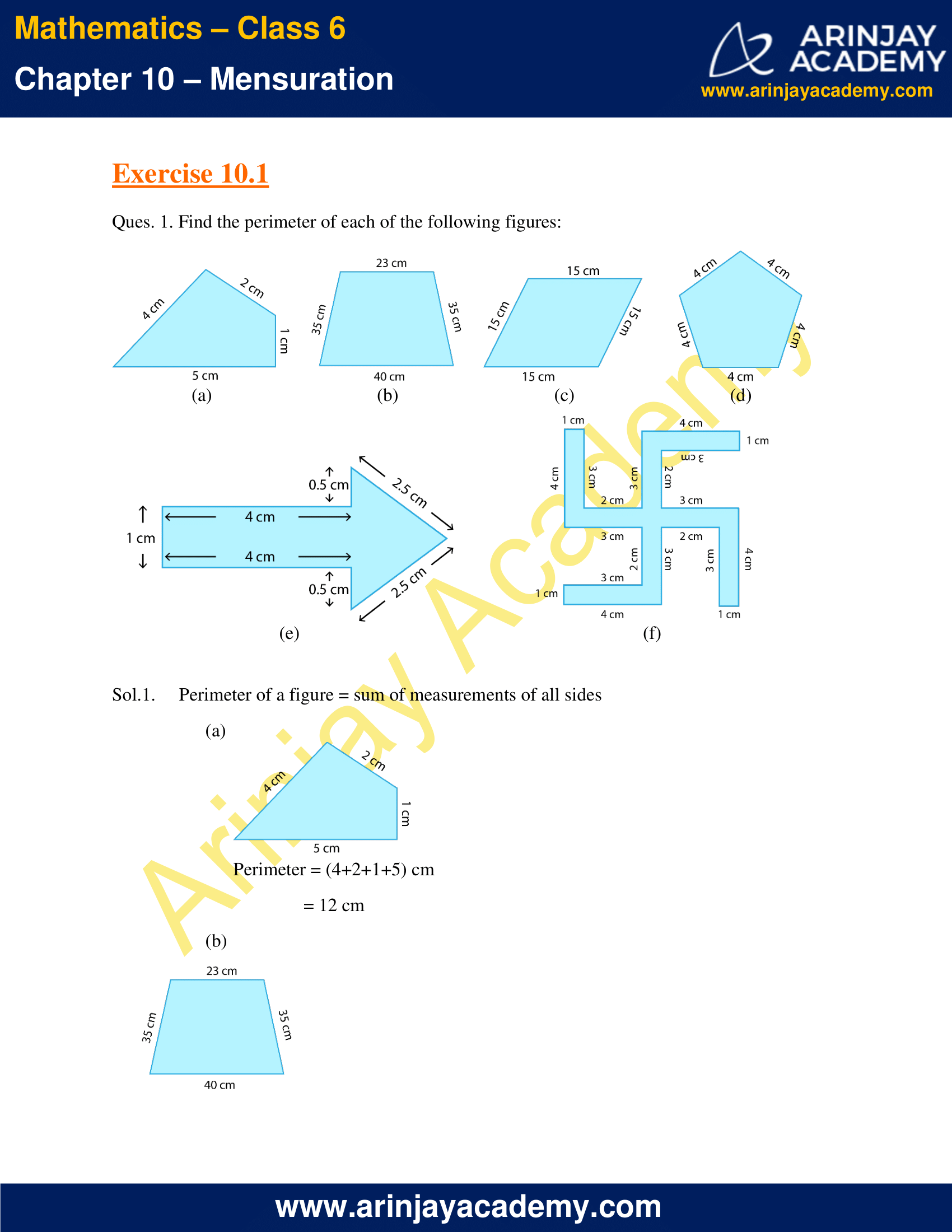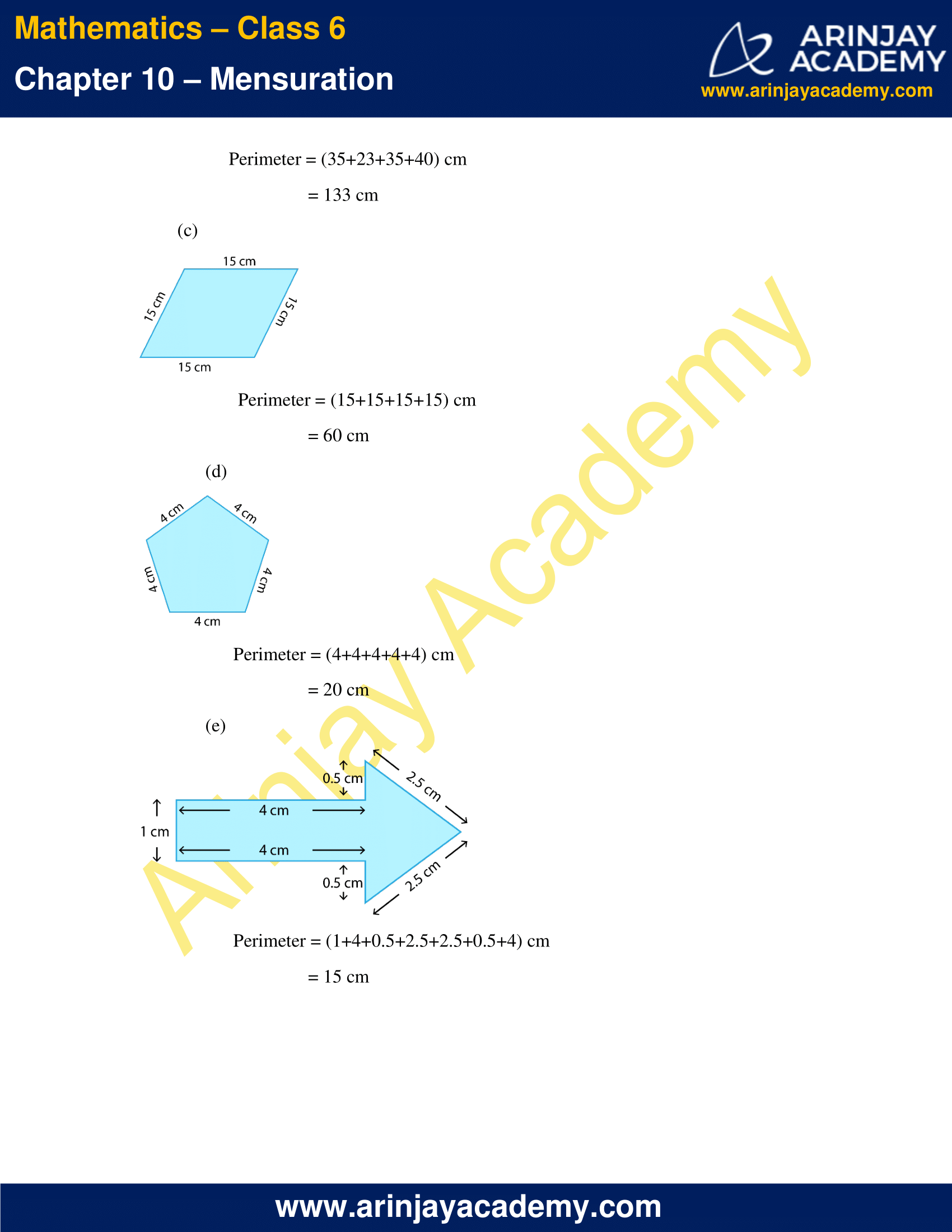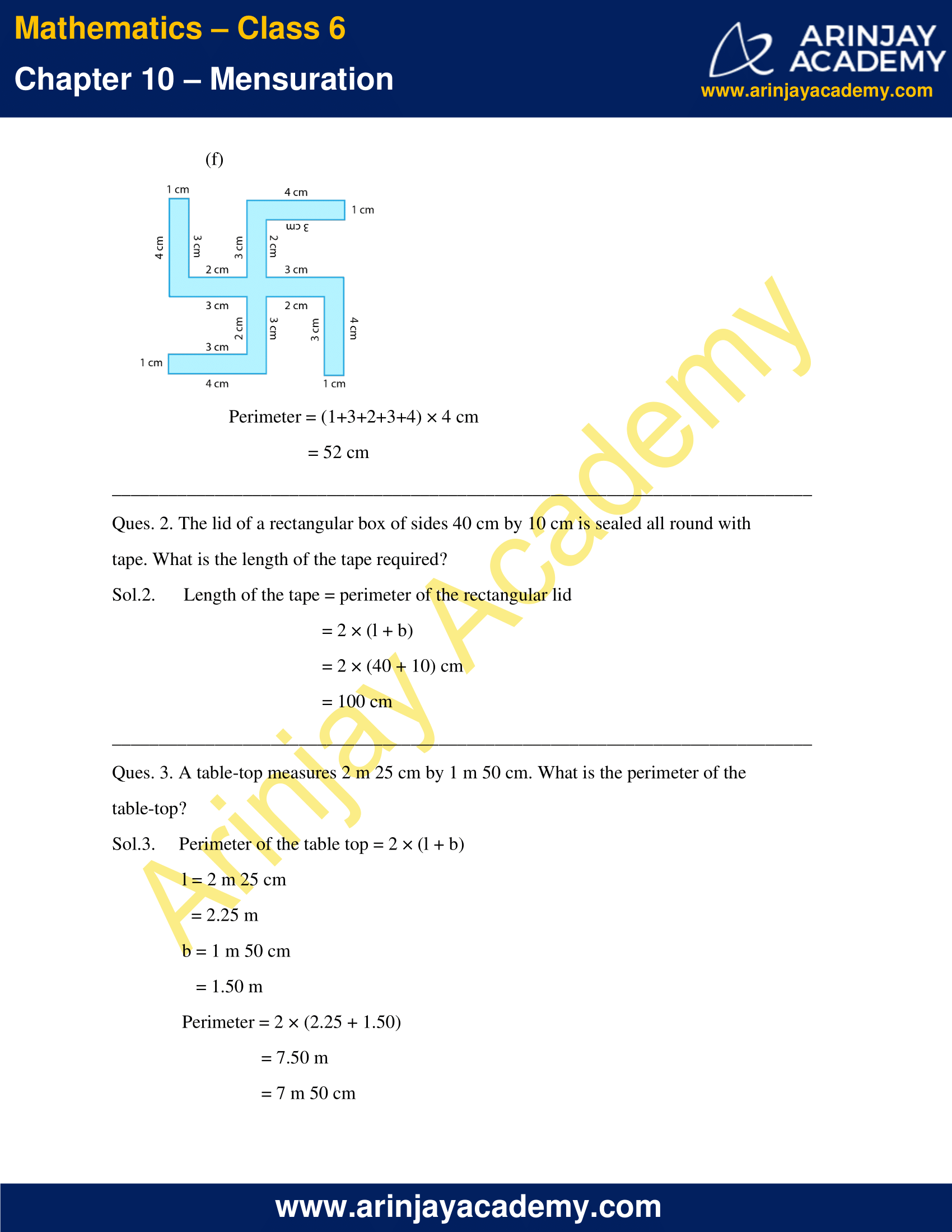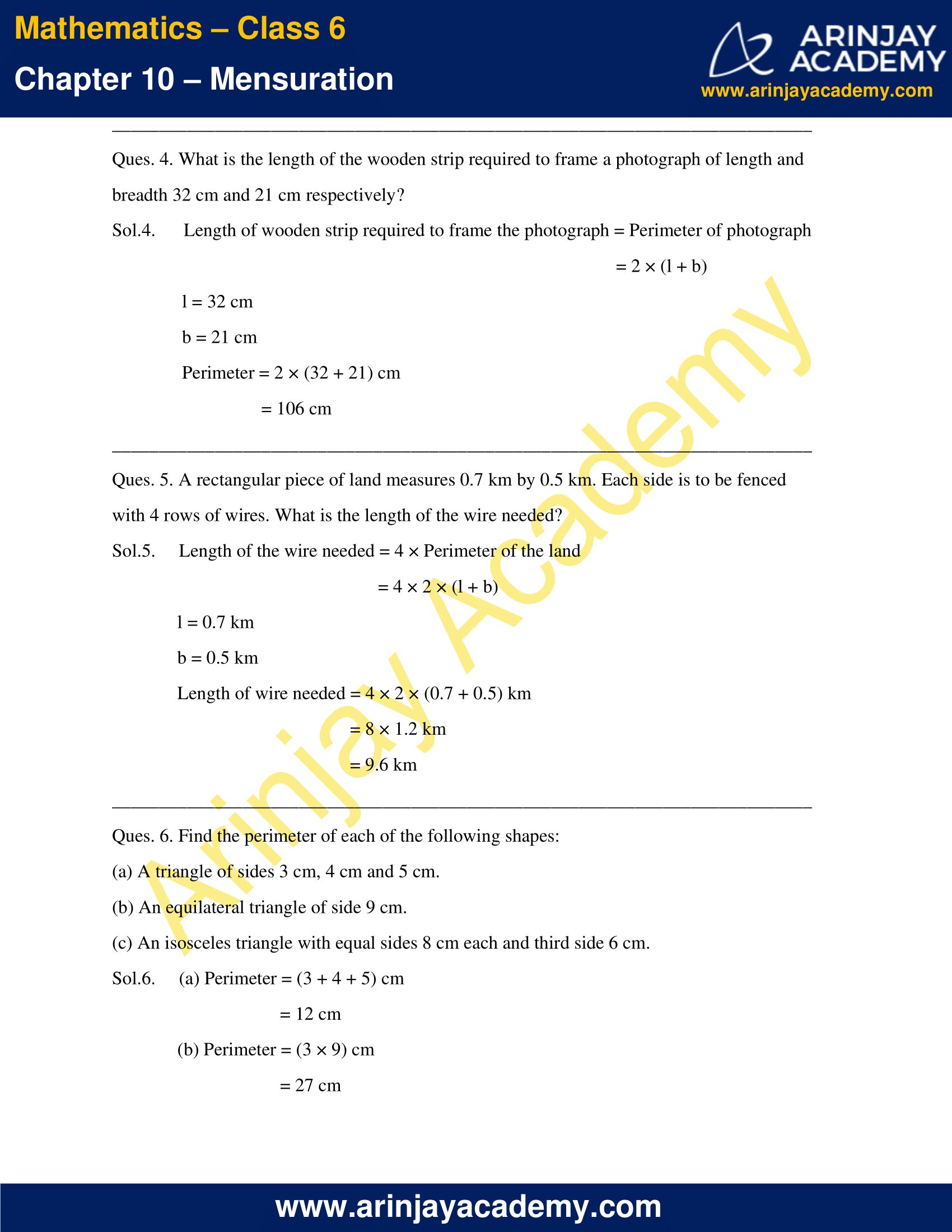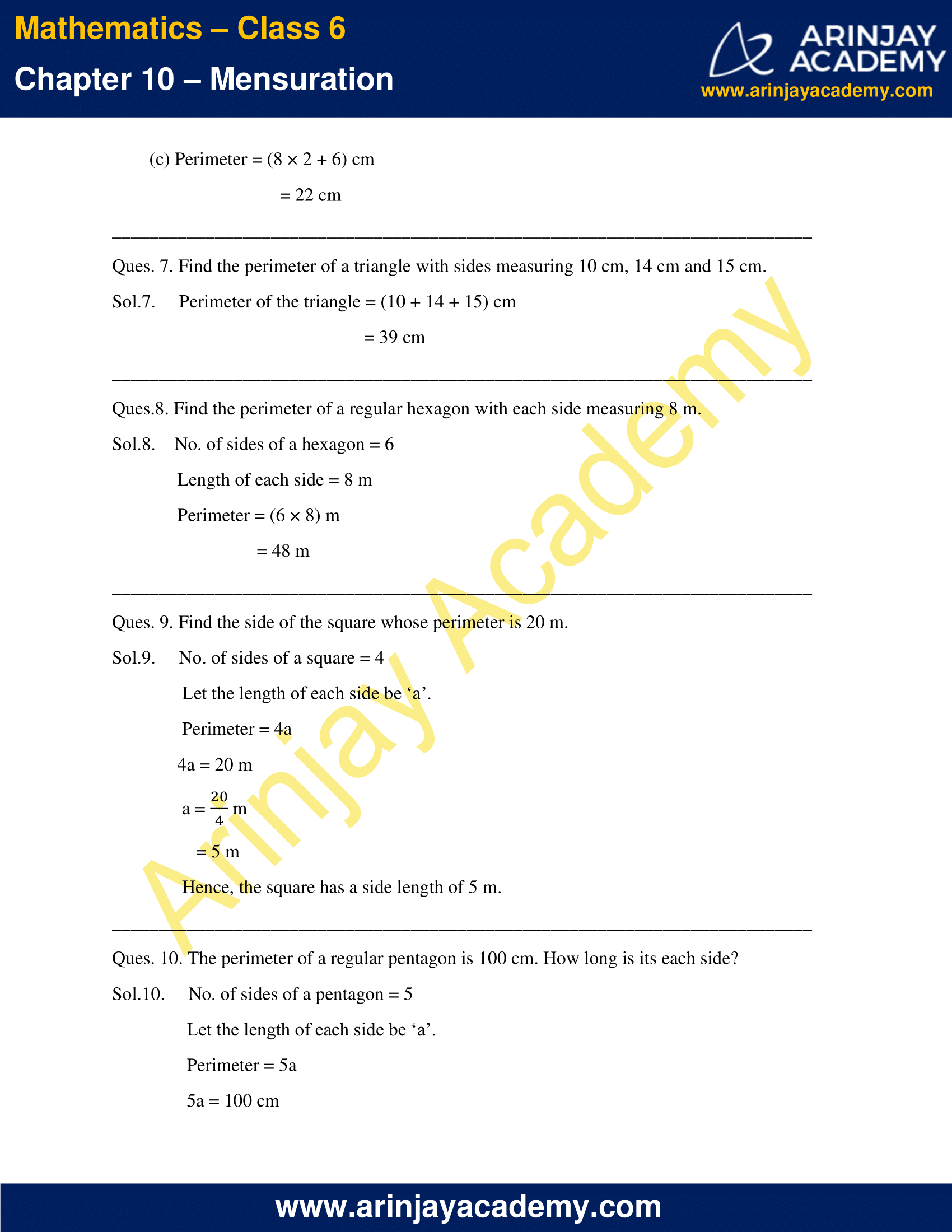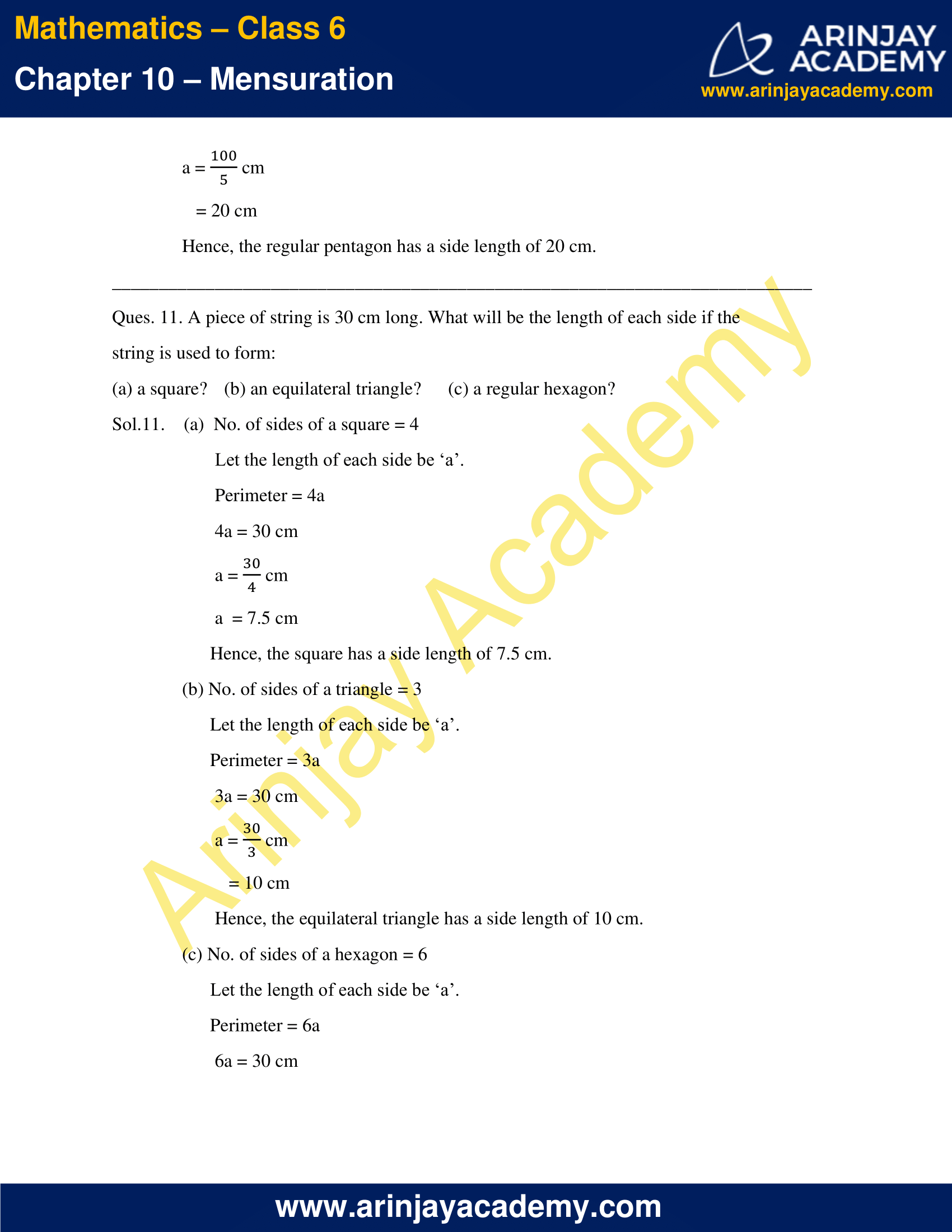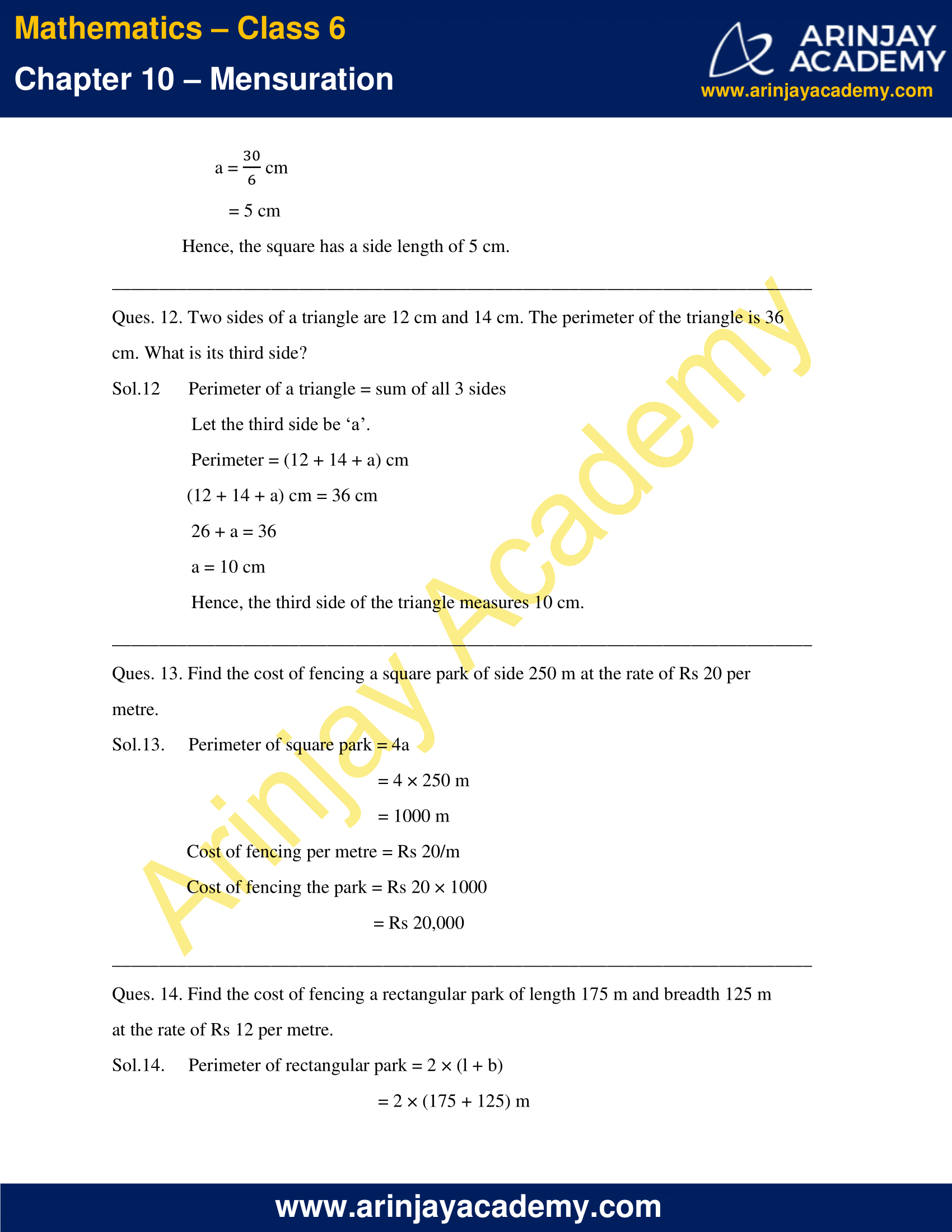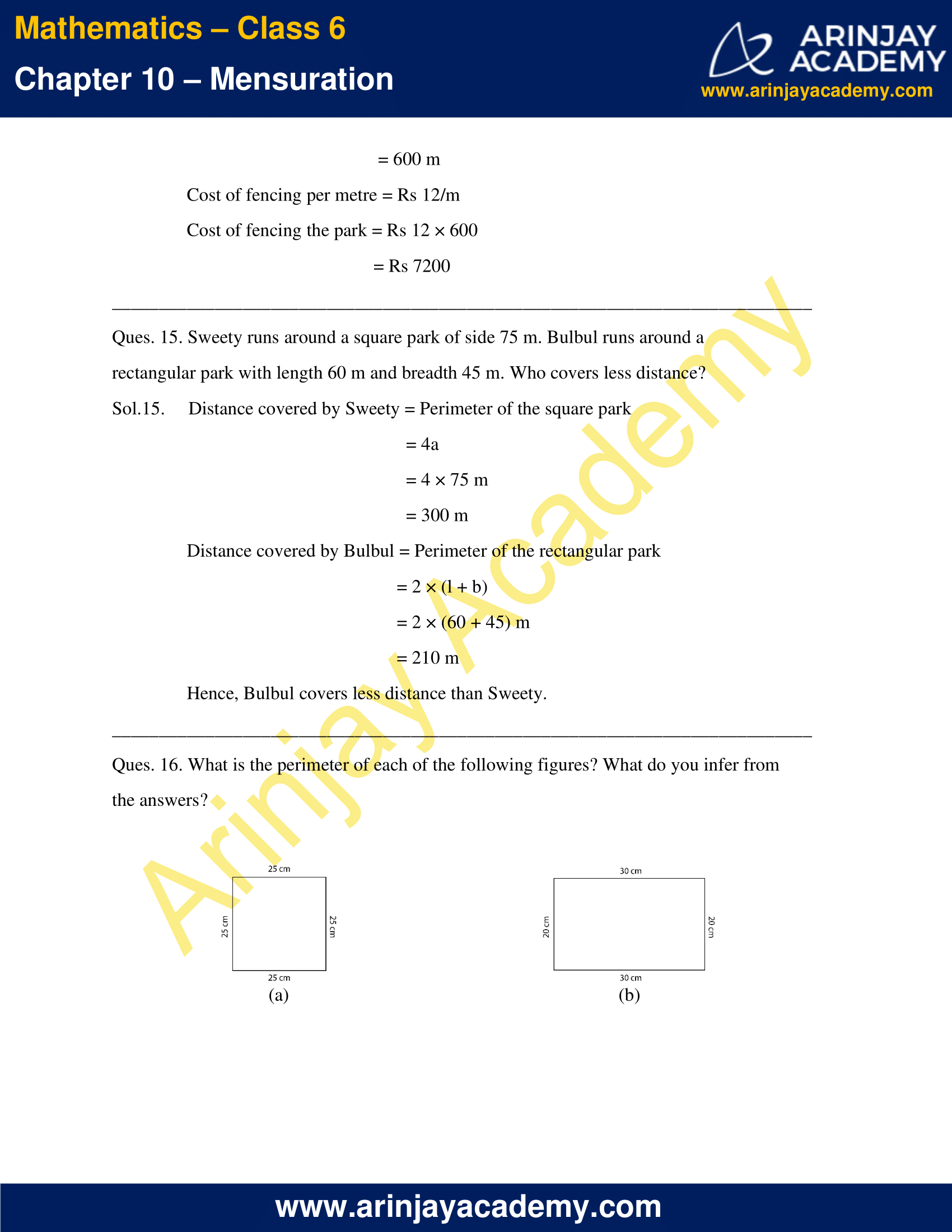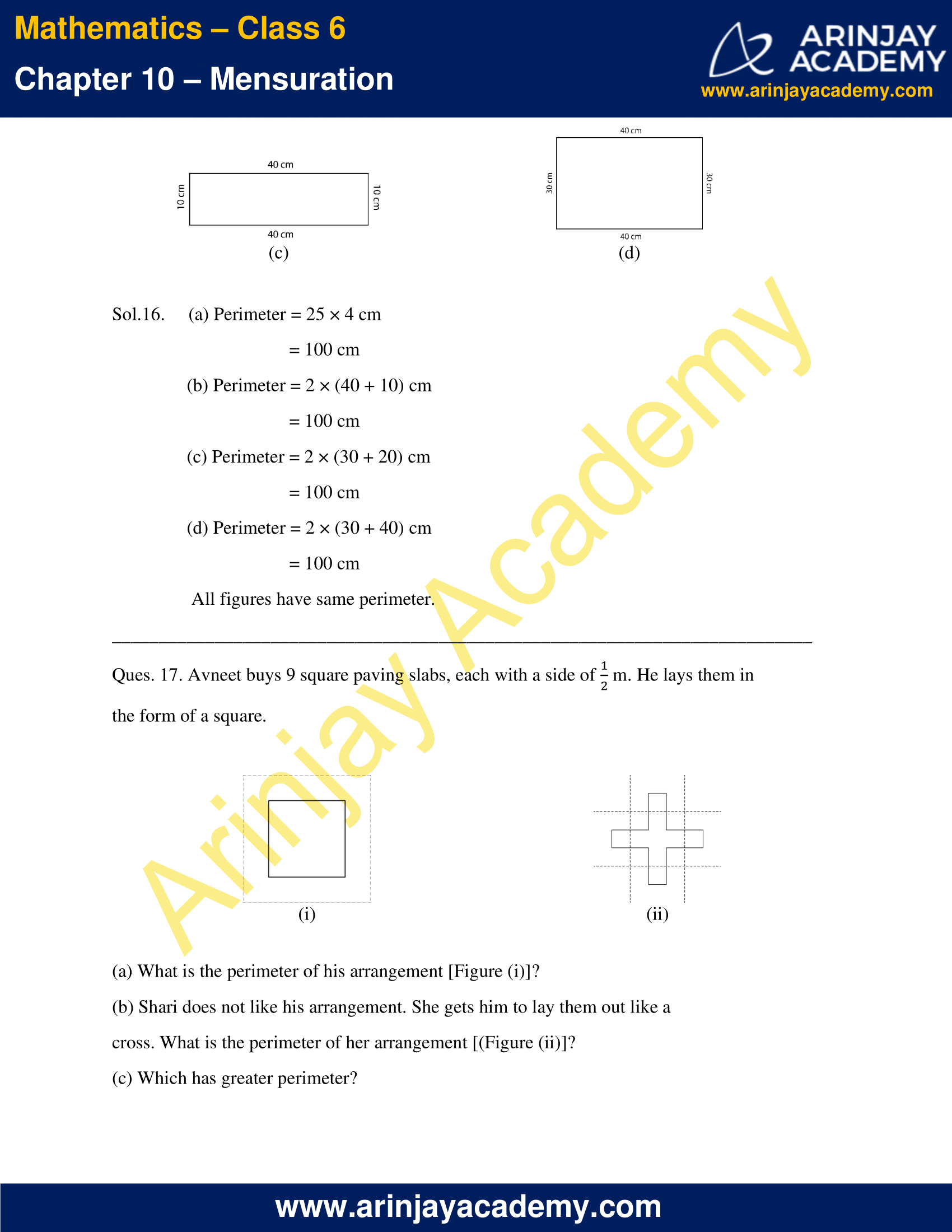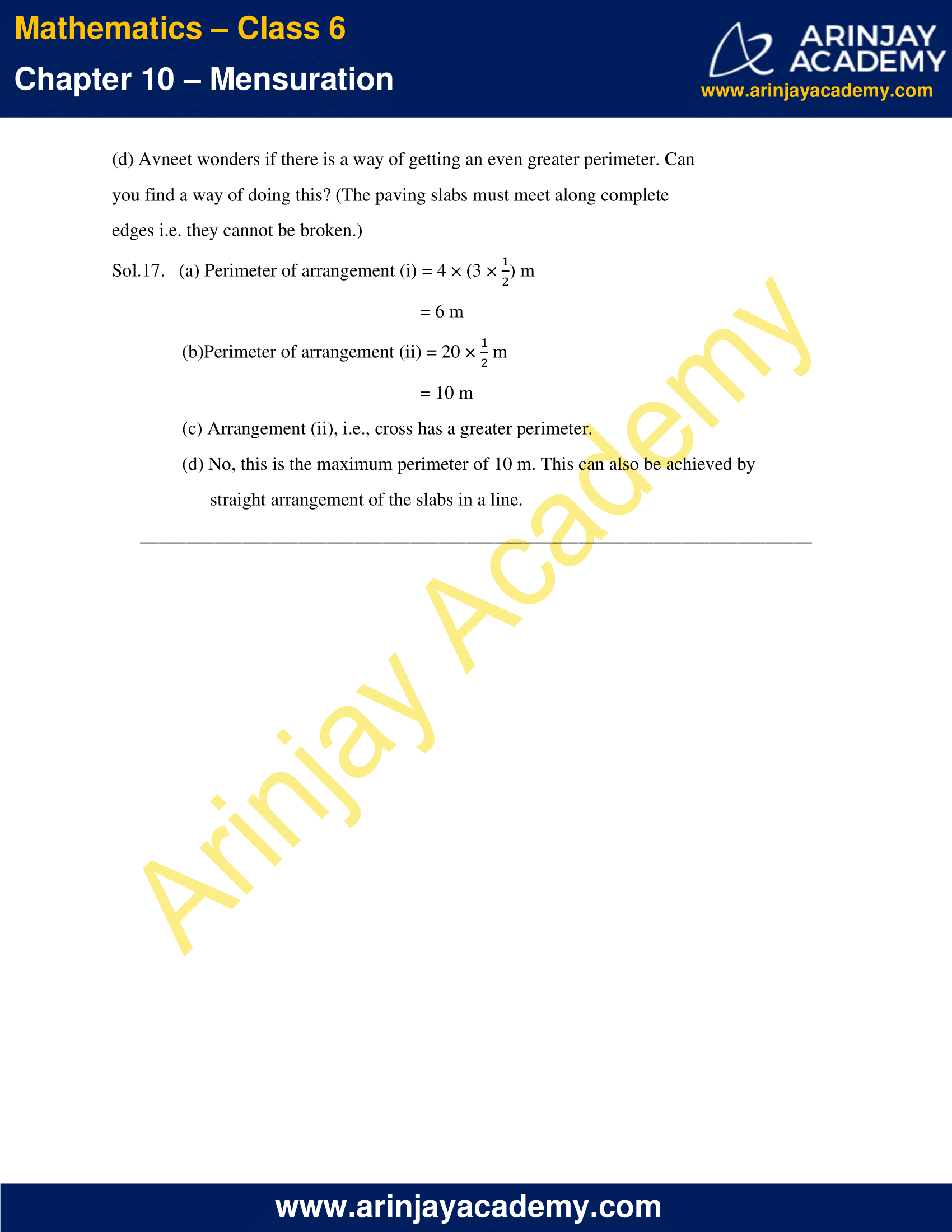### NCERT Solutions for Class 6 Maths Chapter 10 Exercise 10.2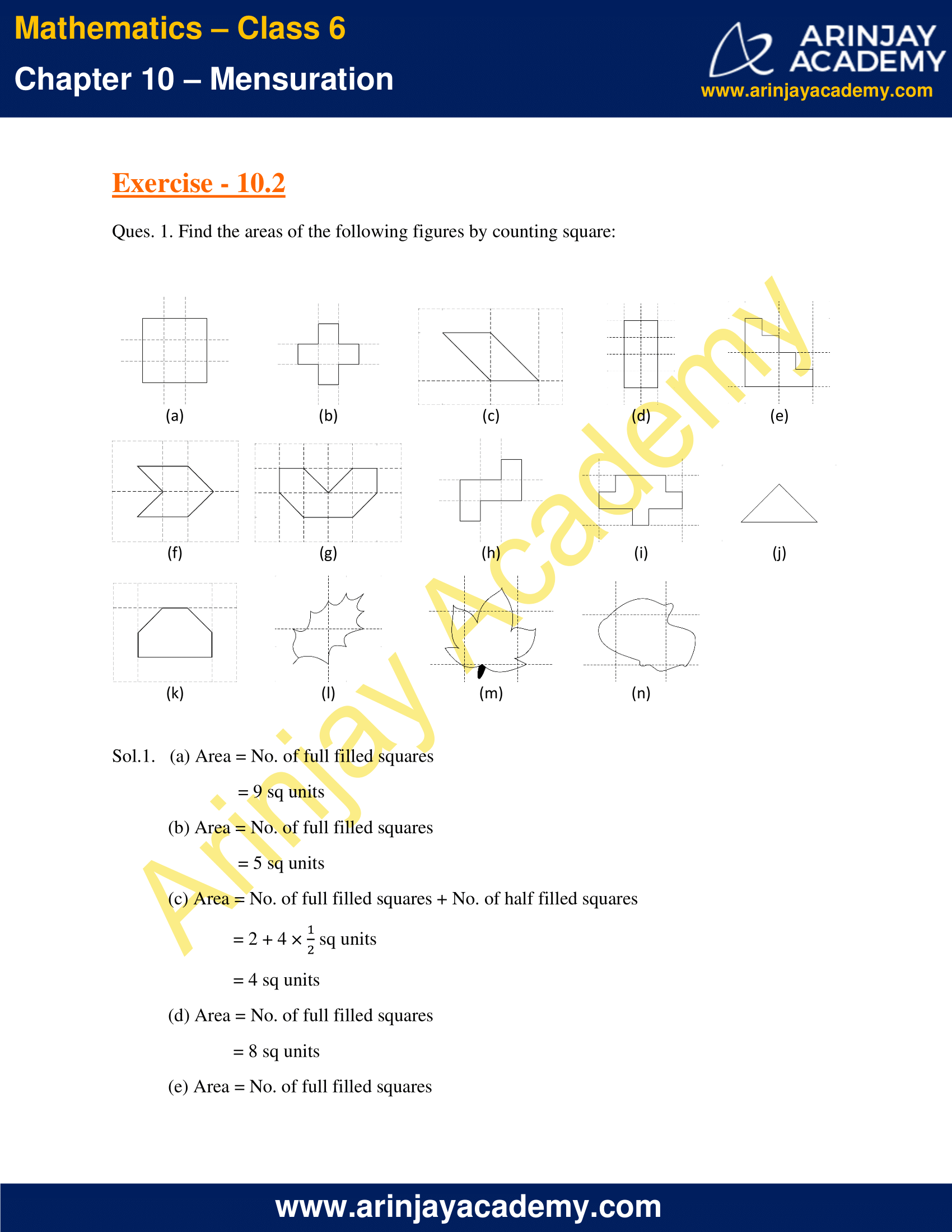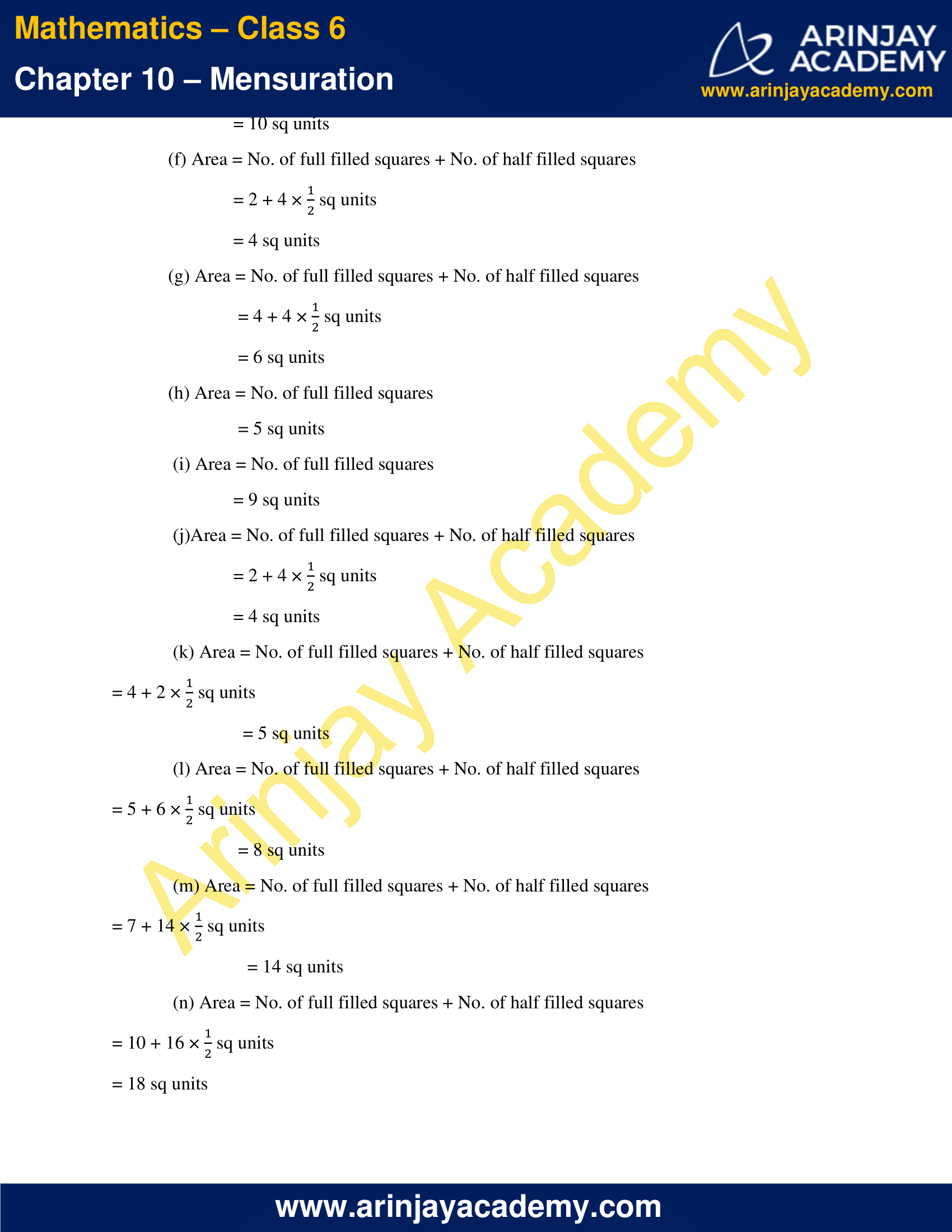### NCERT Solutions for Class 6 Maths Chapter 10 Exercise 10.3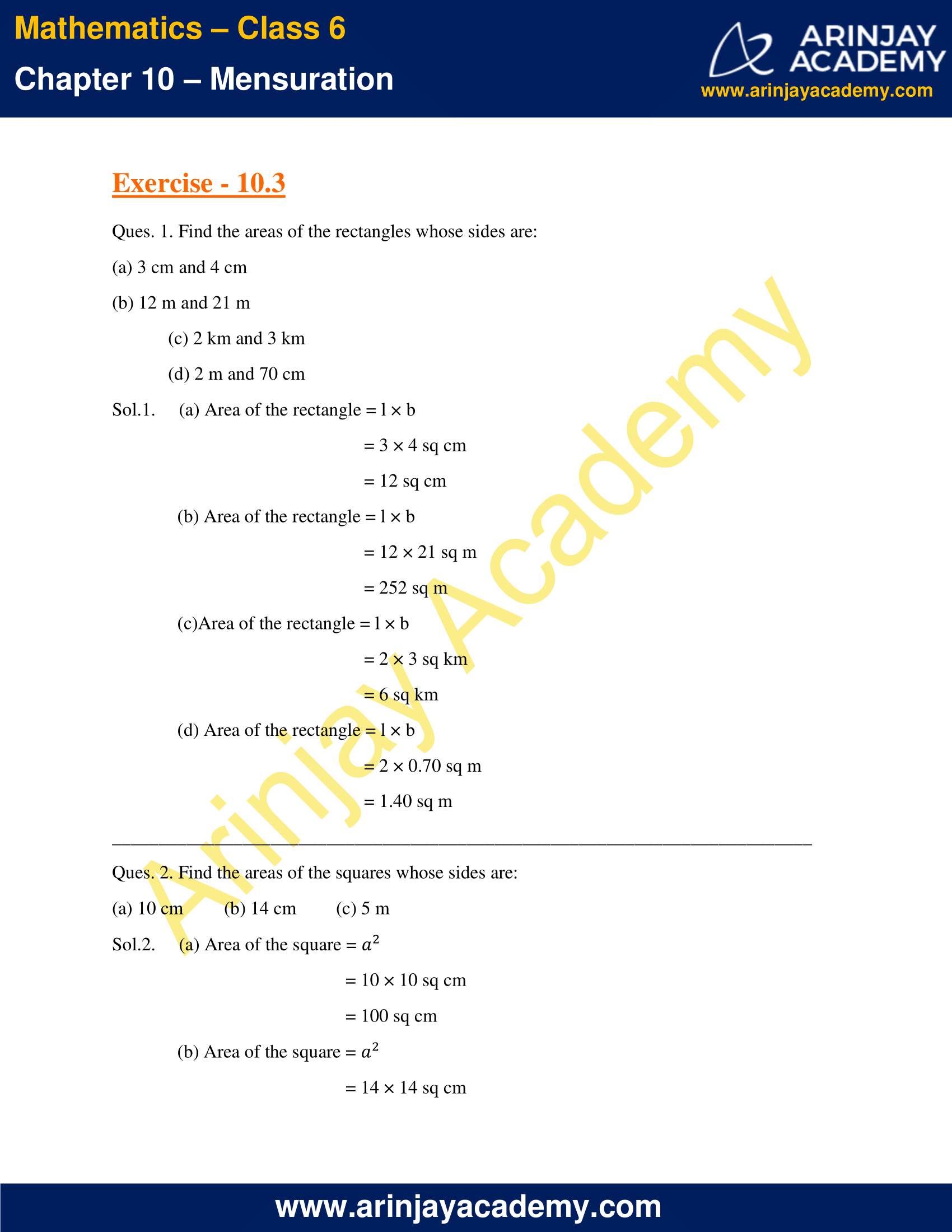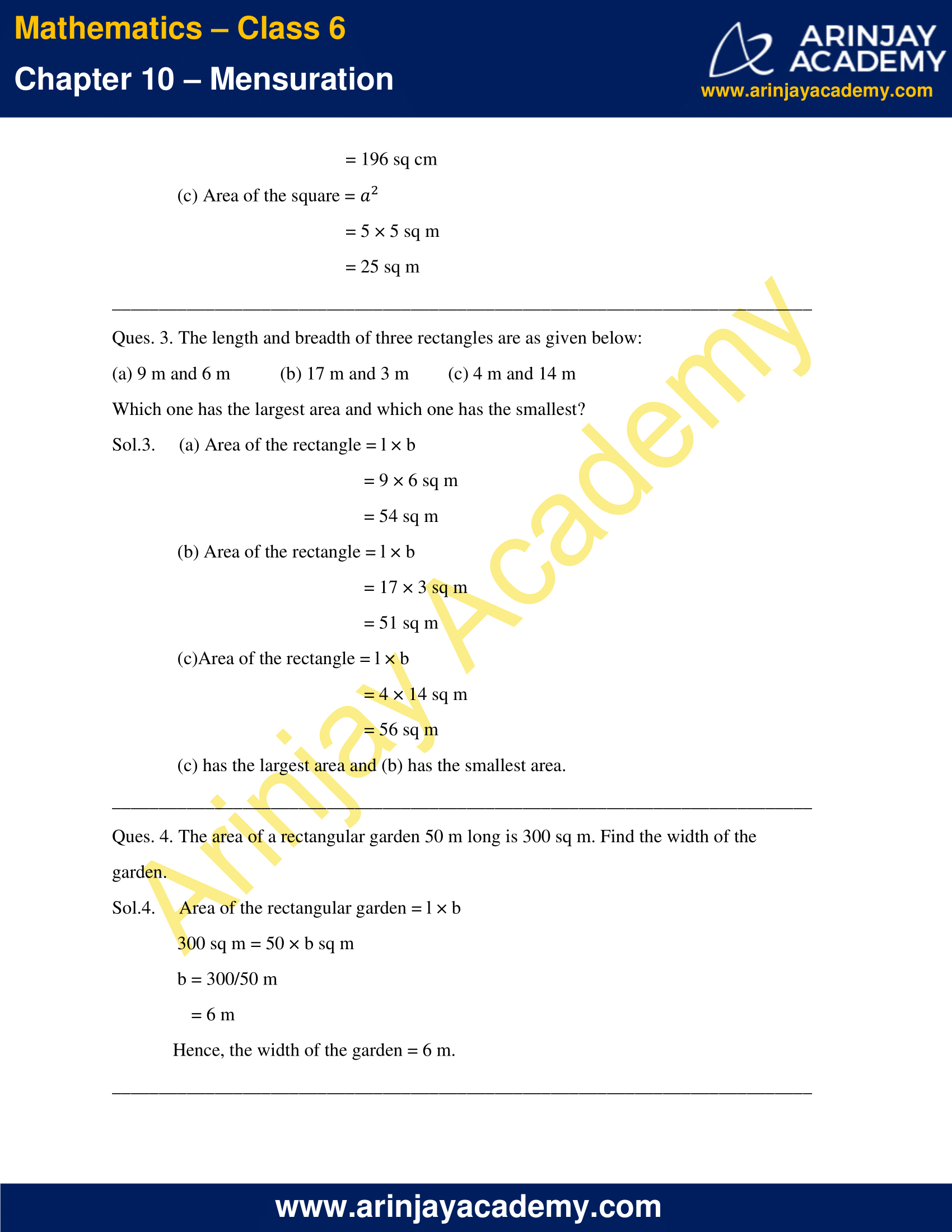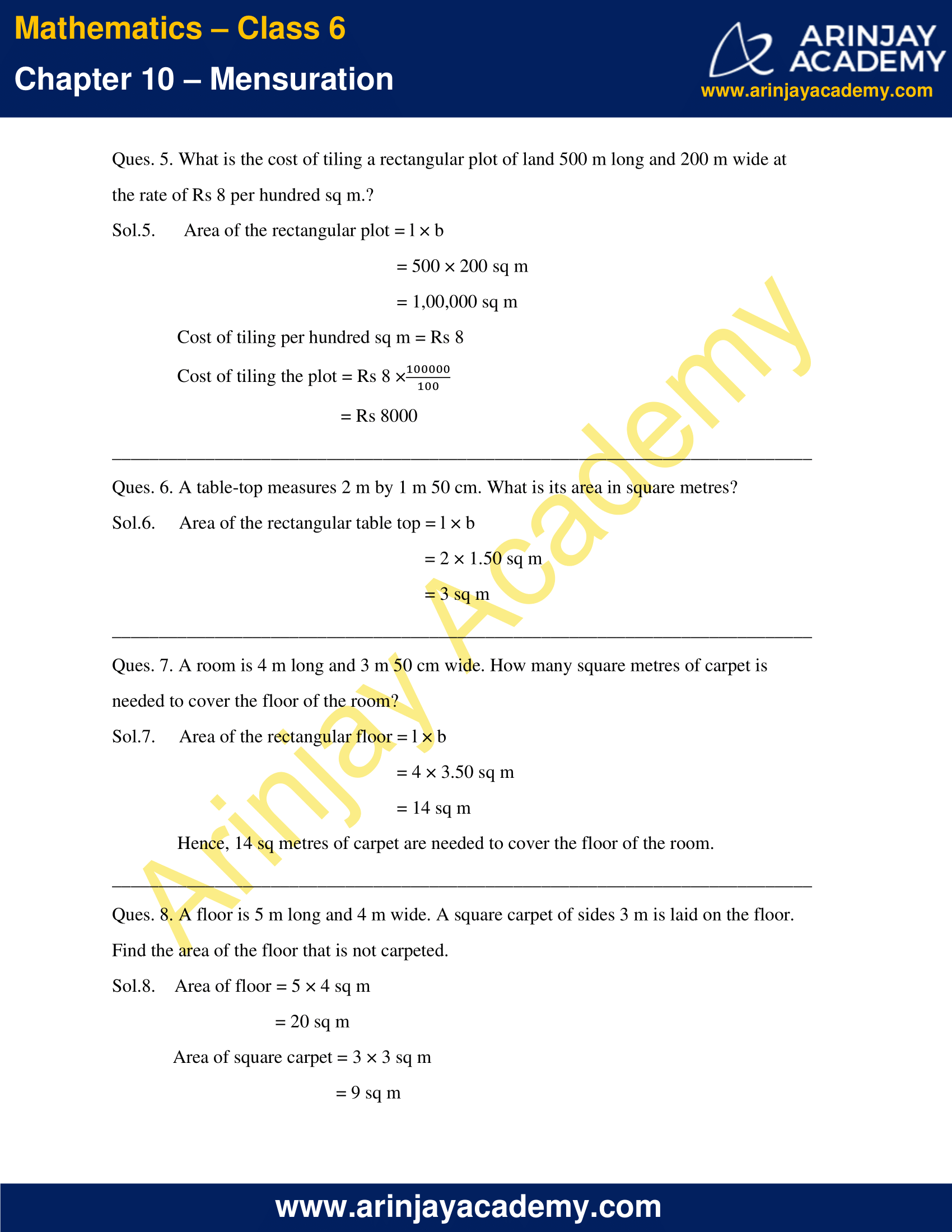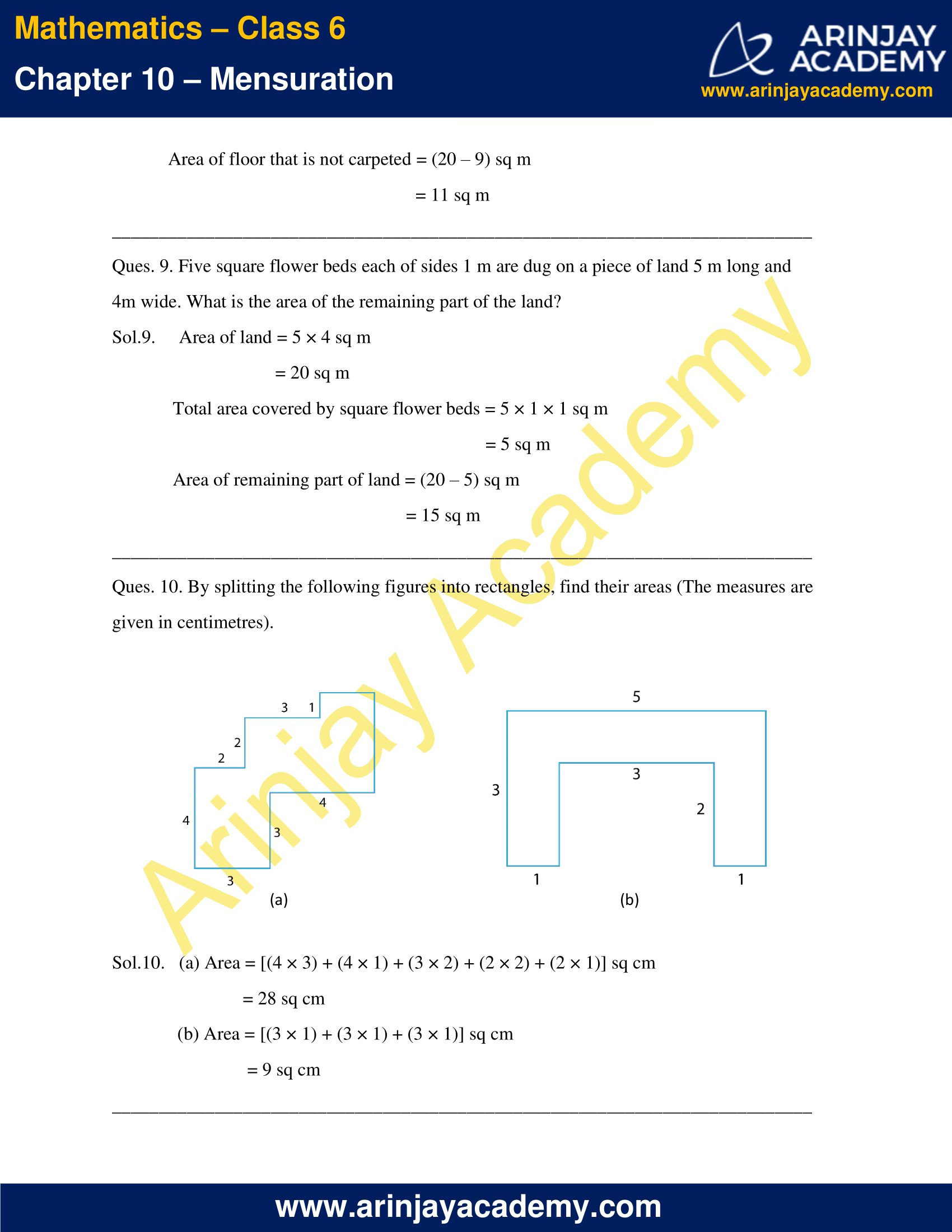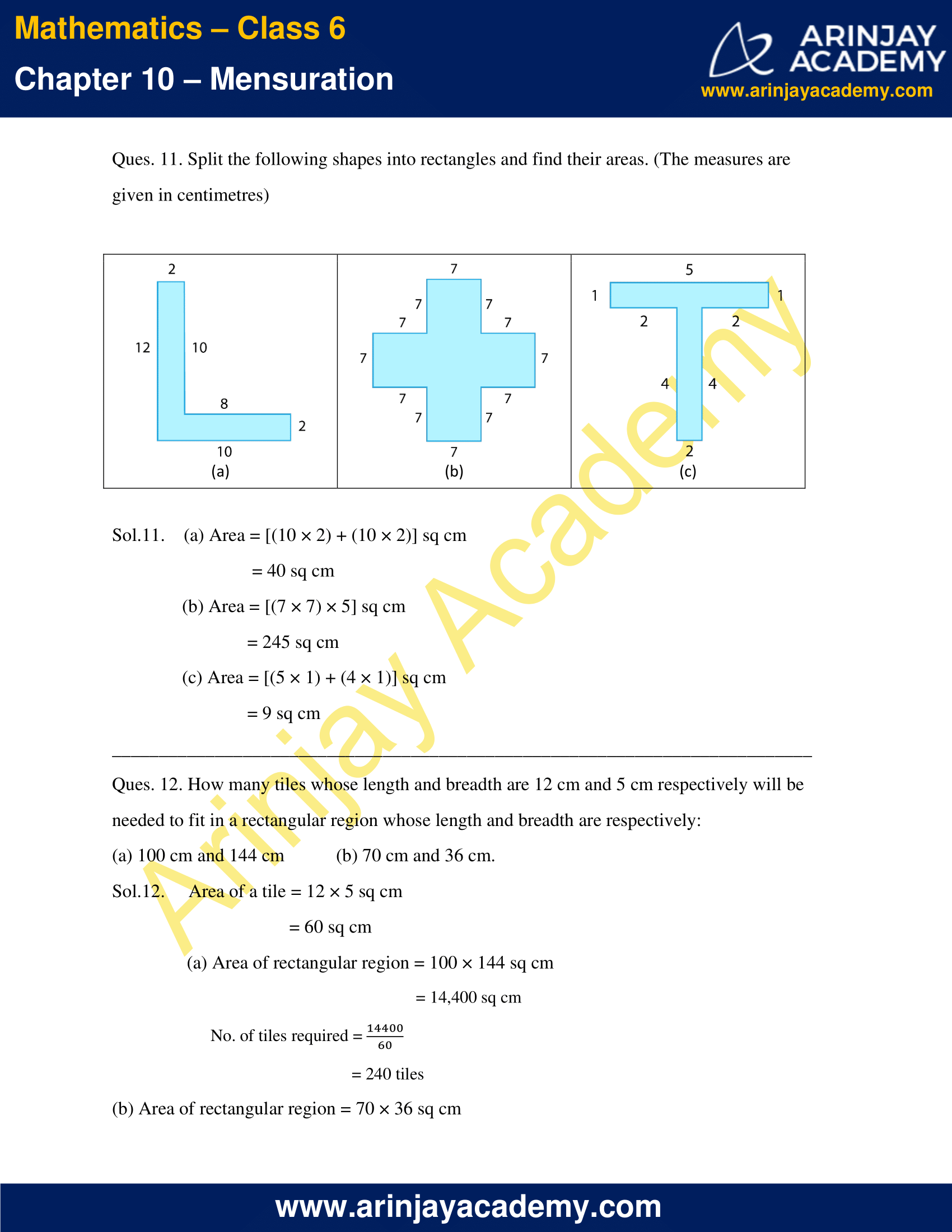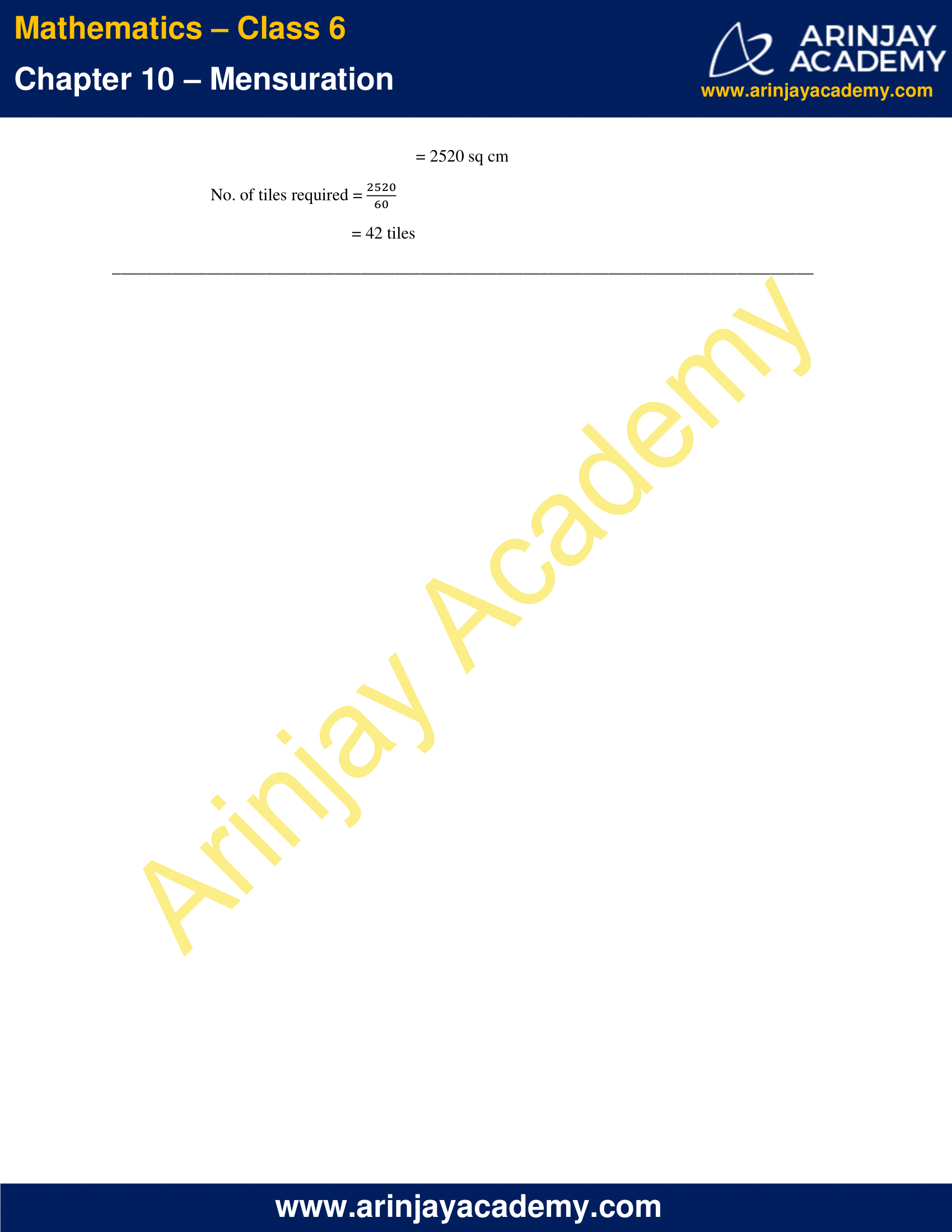### NCERT Solutions for Class 6 Maths Chapter 10 Exercise 10.1

Ques. 1. Find the perimeter of each of the following figures: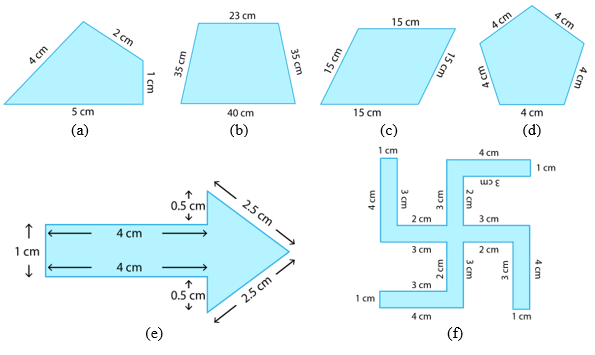Sol.1. Perimeter of a figure = sum of measurements of all sides

a)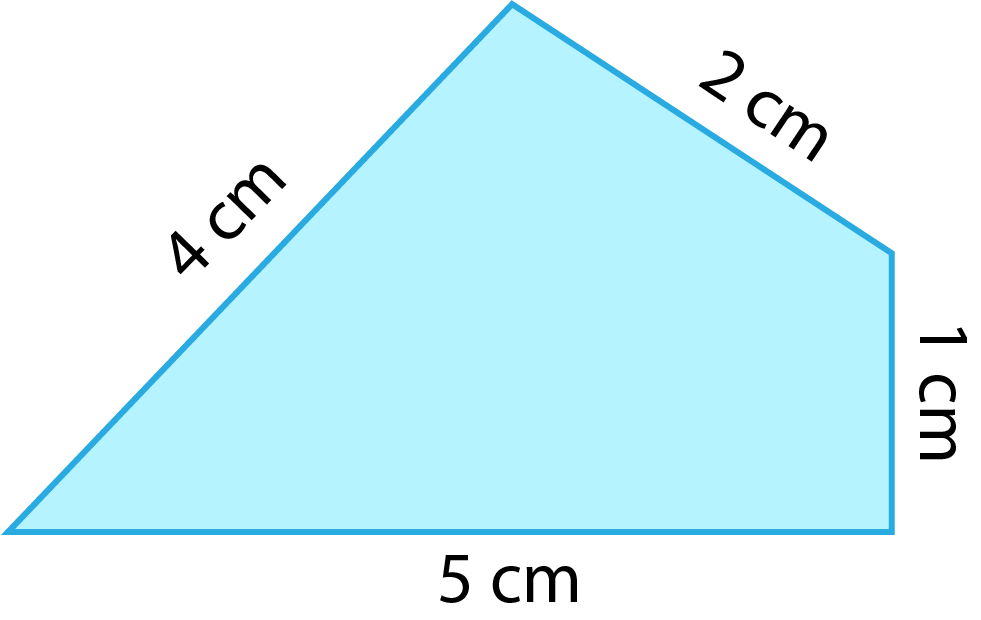Perimeter = (4+2+1+5) cm
= 12 cm

(b)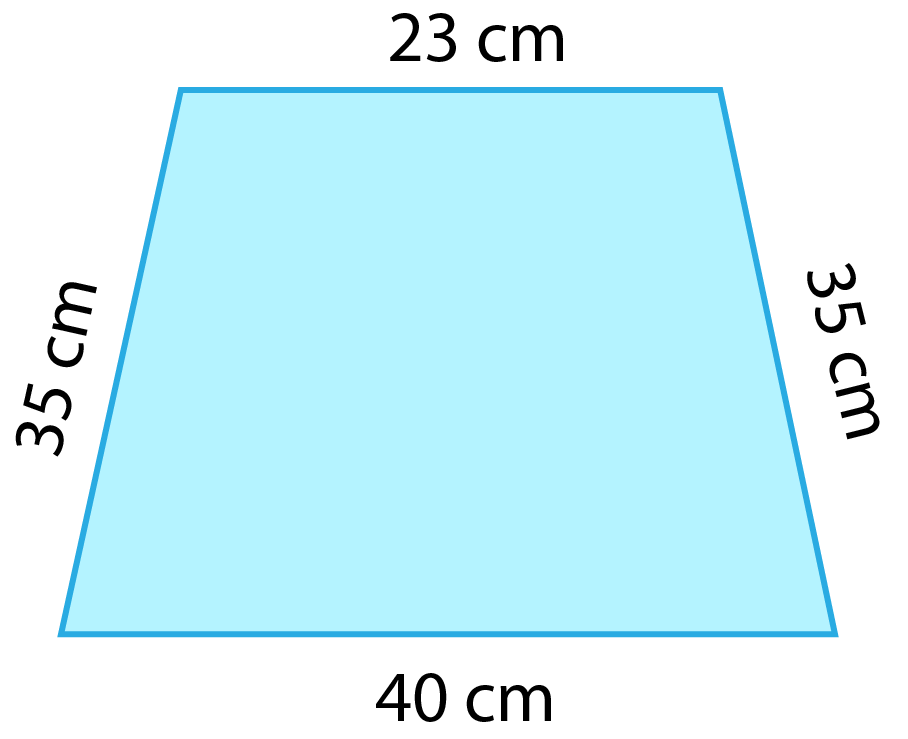Perimeter = (35+23+35+40) cm
= 133 cm

(c)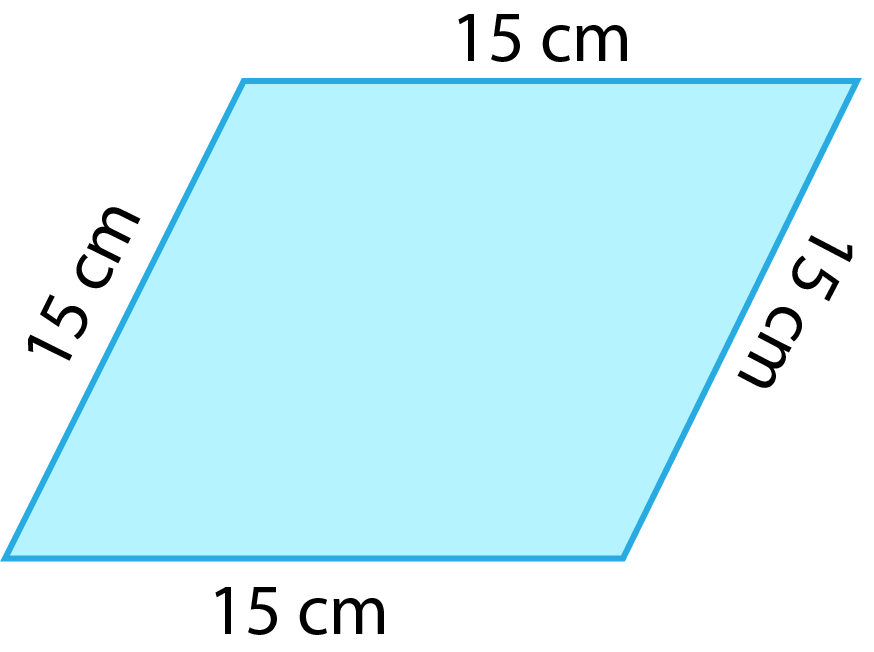Perimeter = (15+15+15+15) cm
= 60 cm

(d)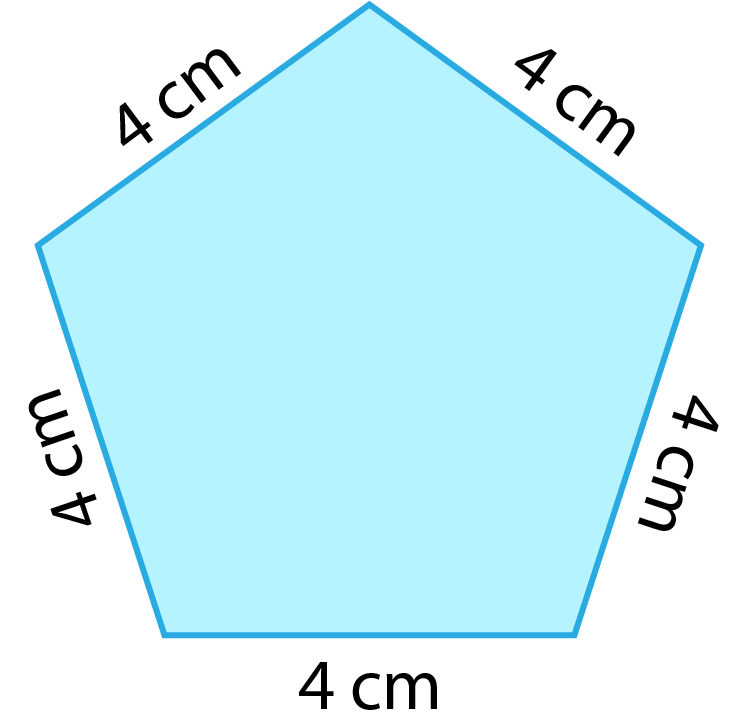Perimeter = (4+4+4+4+4) cm
= 20 cm

(e)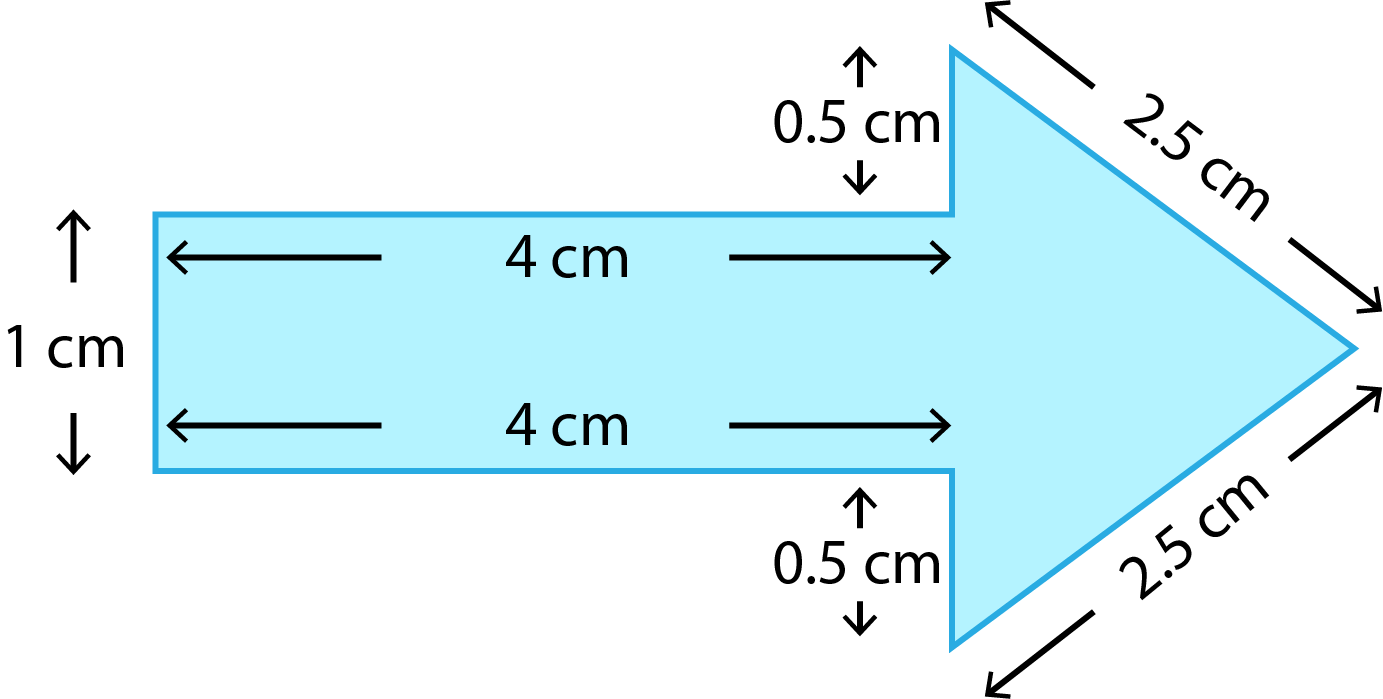Perimeter = (1+4+0.5+2.5+2.5+0.5+4) cm
= 15 cm

(f)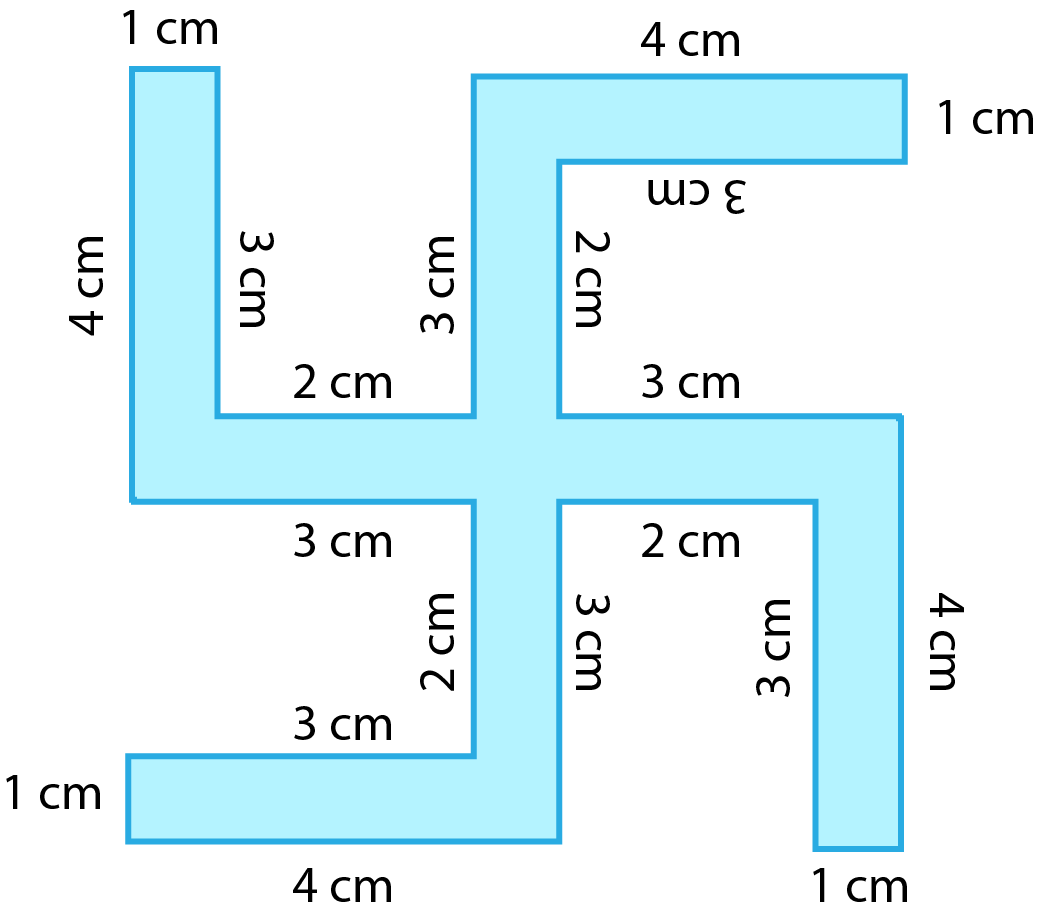Perimeter = (1+3+2+3+4) × 4 cm
= 52 cm

Ques. 2. The lid of a rectangular box of sides 40 cm by 10 cm is sealed all round with tape. What is the length of the tape required?

Sol.2. Length of the tape = perimeter of the rectangular lid
= 2 × (l + b)
= 2 × (40 + 10) cm
= 100 cm

Ques. 3. A table-top measures 2 m 25 cm by 1 m 50 cm. What is the perimeter of the table-top?

Sol.3. Perimeter of the table top = 2 × (l + b)
l = 2 m 25 cm
= 2.25 m
b = 1 m 50 cm
= 1.50 m
Perimeter = 2 × (2.25 + 1.50)
= 7.50 m
= 7 m 50 cm

Ques. 4. What is the length of the wooden strip required to frame a photograph of length and breadth 32 cm and 21 cm respectively?

Sol.4. Length of wooden strip required to frame the photograph = Perimeter of photograph = 2 × (l + b)
l = 32 cm
b = 21 cm
Perimeter = 2 × (32 + 21) cm
= 106 cm

Ques. 5. A rectangular piece of land measures 0.7 km by 0.5 km. Each side is to be fenced with 4 rows of wires. What is the length of the wire needed?

Sol.5. Length of the wire needed = 4 × Perimeter of the land = 4 × 2 × (l + b)
l = 0.7 km
b = 0.5 km
Length of wire needed = 4 × 2 × (0.7 + 0.5) km
= 8 × 1.2 km
= 9.6 km

Ques. 6. Find the perimeter of each of the following shapes:
(a) A triangle of sides 3 cm, 4 cm and 5 cm.

(b) An equilateral triangle of side 9 cm.
(c) An isosceles triangle with equal sides 8 cm each and third side 6 cm.

Sol.6. (a) Perimeter = (3 + 4 + 5) cm
= 12 cm

(b) Perimeter = (3 × 9) cm
= 27 cm

(c) Perimeter = (8 × 2 + 6) cm
= 22 cm

Ques. 7. Find the perimeter of a triangle with sides measuring 10 cm, 14 cm and 15 cm.

Sol.7. Perimeter of the triangle = (10 + 14 + 15) cm
= 39 cm

Ques.8. Find the perimeter of a regular hexagon with each side measuring 8 m.

Sol.8. No. of sides of a hexagon = 6
Length of each side = 8 m
Perimeter = (6 × 8) m
= 48 m

Ques. 9. Find the side of the square whose perimeter is 20 m.

Sol.9. No. of sides of a square = 4
Let the length of each side be ‘a’.
Perimeter = 4a
4a = 20 m
a = 20/4 m
= 5 m
Hence, the square has a side length of 5 m.

Ques. 10. The perimeter of a regular pentagon is 100 cm. How long is its each side?
Sol.10.
No. of sides of a pentagon = 5
Let the length of each side be ‘a’.
Perimeter = 5a
5a = 100 cm
a = 100/5 cm
= 20 cm
Hence, the regular pentagon has a side length of 20 cm.

Ques. 11. A piece of string is 30 cm long. What will be the length of each side if the string is used to form:
(a) a square?

(b) an equilateral triangle?
(c) a regular hexagon?

Sol.11. (a)  No. of sides of a square = 4
Let the length of each side be ‘a’.
Perimeter = 4a
4a = 30 cm
a = 30/4 cm
a  = 7.5 cm
Hence, the square has a side length of 7.5 cm.

(b) No. of sides of a triangle = 3
Let the length of each side be ‘a’.
Perimeter = 3a
3a = 30 cm
a = 30/3 cm
= 10 cm
Hence, the equilateral triangle has a side length of 10 cm.

(c) No. of sides of a hexagon = 6
Let the length of each side be ‘a’.
Perimeter = 6a
6a = 30 cm
a = 30/6 cm
= 5 cm
Hence, the square has a side length of 5 cm.

Ques. 12. Two sides of a triangle are 12 cm and 14 cm. The perimeter of the triangle is 36 cm. What is its third side?

Sol.12 Perimeter of a triangle = sum of all 3 sides
Let the third side be ‘a’.
Perimeter = (12 + 14 + a) cm
(12 + 14 + a) cm = 36 cm
26 + a = 36
a = 10 cm
Hence, the third side of the triangle measures 10 cm.

Ques. 13. Find the cost of fencing a square park of side 250 m at the rate of Rs 20 per metre.

Sol.13. Perimeter of square park = 4a
= 4 × 250 m
= 1000 m

Cost of fencing per metre = Rs 20/m
Cost of fencing the park = Rs 20 × 1000
= Rs 20,000

Ques. 14. Find the cost of fencing a rectangular park of length 175 m and breadth 125 m at the rate of Rs 12 per metre.

Sol.14. Perimeter of rectangular park = 2 × (l + b)
= 2 × (175 + 125) m
= 600 m
Cost of fencing per metre = Rs 12/m
Cost of fencing the park = Rs 12 × 600
= Rs 7200

Ques. 15. Sweety runs around a square park of side 75 m. Bulbul runs around a rectangular park with length 60 m and breadth 45 m. Who covers less distance?

Sol.15. Distance covered by Sweety = Perimeter of the square park
= 4a
= 4 × 75 m
= 300 m
Distance covered by Bulbul = Perimeter of the rectangular park
= 2 × (l + b)
= 2 × (60 + 45) m
= 210 m
Hence, Bulbul covers less distance than Sweety.

Ques. 16. What is the perimeter of each of the following figures? What do you infer from the answers?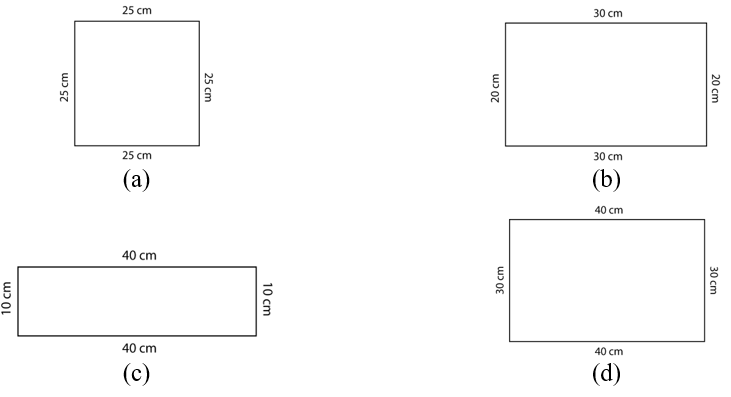Sol.16.

(a) Perimeter = 25 × 4 cm
= 100 cm

(b) Perimeter = 2 × (40 + 10) cm
= 100 cm

(c) Perimeter = 2 × (30 + 20) cm
= 100 cm

(d) Perimeter = 2 × (30 + 40) cm
= 100 cm

All figures have same perimeter.

Ques. 17. Avneet buys 9 square paving slabs, each with a side of  m. He lays them in the form of a square.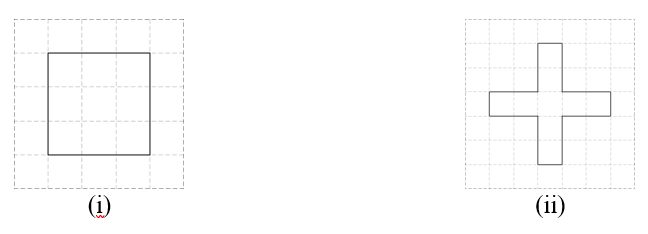(a) What is the perimeter of his arrangement [Figure (i)]?
(b) Shari does not like his arrangement. She gets him to lay them out like a cross. What is the perimeter of her arrangement [(Figure (ii)]?
(c) Which has greater perimeter?
(d) Avneet wonders if there is a way of getting an even greater perimeter. Can you find a way of doing this? (The paving slabs must meet along complete edges i.e. they cannot be broken.)

Sol.17.

(a) Perimeter of arrangement (i) = 4 × (3 × 1/2) m
= 6 m

(b) Perimeter of arrangement (ii) = 20 × 1/2 m
= 10 m

(c) Arrangement (ii), i.e., cross has a greater perimeter.

(d) No, this is the maximum perimeter of 10 m. This can also be achieved by straight arrangement of the slabs in a line.

### NCERT Solutions for Class 6 Maths Chapter 10 Exercise 10.2

Ques. 1. Find the areas of the following figures by counting square: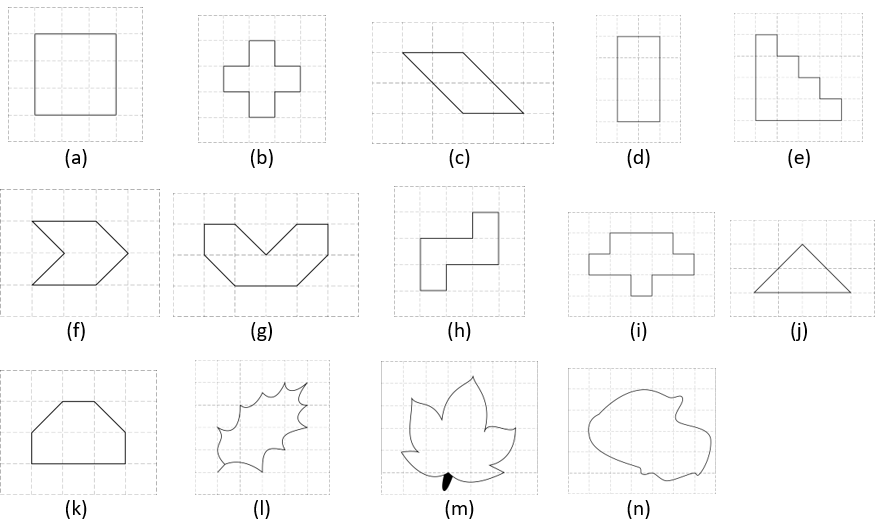Sol.1.

(a) Area = No. of full filled squares
= 9 sq units

(b) Area = No. of full filled squares
= 5 sq units

(c) Area = No. of full filled squares + No. of half filled squares
= 2 + 4 × 1/2 sq units
= 4 sq units

(d) Area = No. of full filled squares
= 8 sq units

(e) Area = No. of full filled squares
= 10 sq units

(f) Area = No. of full filled squares + No. of half filled squares
= 2 + 4 × 1/2 sq units
= 4 sq units

(g) Area = No. of full filled squares + No. of half filled squares
= 4 + 4 × 1/2 sq units
= 6 sq units

(h) Area = No. of full filled squares
= 5 sq units

(i) Area = No. of full filled squares
= 9 sq units

(j) Area = No. of full filled squares + No. of half filled squares
= 2 + 4 × 1/2 sq units
= 4 sq units

(k) Area = No. of full filled squares + No. of half filled squares
= 4 + 2 × 1/2 sq units
= 5 sq units

(l) Area = No. of full filled squares + No. of half filled squares
= 5 + 6 × 1/2 sq units
= 8 sq units

(m) Area = No. of full filled squares + No. of half filled squares
= 7 + 14 × 1/2 sq units
= 14 sq units

(n) Area = No. of full filled squares + No. of half filled squares
= 10 + 16 × 1/2 sq units
= 18 sq units

### NCERT Solutions for Class 6 Maths Chapter 10 Exercise 10.3

Ques. 1. Find the areas of the rectangles whose sides are:
(a) 3 cm and 4 cm
(b) 12 m and 21 m
(c) 2 km and 3 km
(d) 2 m and 70 cm

Sol.1.

(a) Area of the rectangle = l × b
= 3 × 4 sq cm
= 12 sq cm

(b) Area of the rectangle = l × b
= 12 × 21 sq m
= 252 sq m

(c) Area of the rectangle = l × b
= 2 × 3 sq km
= 6 sq km

(d) Area of the rectangle = l × b
= 2 × 0.70 sq m
= 1.40 sq m

Ques. 2. Find the areas of the squares whose sides are:
(a) 10 cm
(b) 14 cm
(c) 5 m

Sol.2.

(a) Area of the square = a²
= 10 × 10 sq cm
= 100 sq cm

(b) Area of the square = a²
= 14 × 14 sq cm
= 196 sq cm

(c) Area of the square = a²
= 5 × 5 sq m
= 25 sq m

Ques. 3. The length and breadth of three rectangles are as given below:
(a) 9 m and 6 m
(b) 17 m and 3 m
(c) 4 m and 14 m
Which one has the largest area and which one has the smallest?

Sol.3.

(a) Area of the rectangle = l × b
= 9 × 6 sq m
= 54 sq m

(b) Area of the rectangle = l × b
= 17 × 3 sq m
= 51 sq m

(c) Area of the rectangle = l × b
= 4 × 14 sq m
= 56 sq m

(c) has the largest area and (b) has the smallest area.

Ques. 4. The area of a rectangular garden 50 m long is 300 sq m. Find the width of the garden.

Sol.4. Area of the rectangular garden = l × b
300 sq m = 50 × b sq m
b = 300/50 m
= 6 m
Hence, the width of the garden = 6 m.

Ques. 5. What is the cost of tiling a rectangular plot of land 500 m long and 200 m wide at the rate of Rs 8 per hundred sq m.?

Sol.5. Area of the rectangular plot = l × b
= 500 × 200 sq m
= 1,00,000 sq m
Cost of tiling per hundred sq m = Rs 8
Cost of tiling the plot = Rs 8 × 100000/100
= Rs 8000

Ques. 6. A table-top measures 2 m by 1 m 50 cm. What is its area in square metres?

Sol.6. Area of the rectangular table top = l × b
= 2 × 1.50 sq m
= 3 sq m

Ques. 7. A room is 4 m long and 3 m 50 cm wide. How many square metres of carpet is needed to cover the floor of the room?

Sol.7. Area of the rectangular floor = l × b
= 4 × 3.50 sq m
= 14 sq m
Hence, 14 sq metres of carpet are needed to cover the floor of the room.

Ques. 8. A floor is 5 m long and 4 m wide. A square carpet of sides 3 m is laid on the floor. Find the area of the floor that is not carpeted.

Sol.8. Area of floor = 5 × 4 sq m
= 20 sq m
Area of square carpet = 3 × 3 sq m
= 9 sq m
Area of floor that is not carpeted = (20 – 9) sq m
= 11 sq m

Ques. 9. Five square flower beds each of sides 1 m are dug on a piece of land 5 m long and 4m wide. What is the area of the remaining part of the land?

Sol.9. Area of land = 5 × 4 sq m
= 20 sq m
Total area covered by square flower beds = 5 × 1 × 1 sq m
= 5 sq m
Area of remaining part of land = (20 – 5) sq m
= 15 sq m

Ques. 10. By splitting the following figures into rectangles, find their areas (The measures are given in centimetres).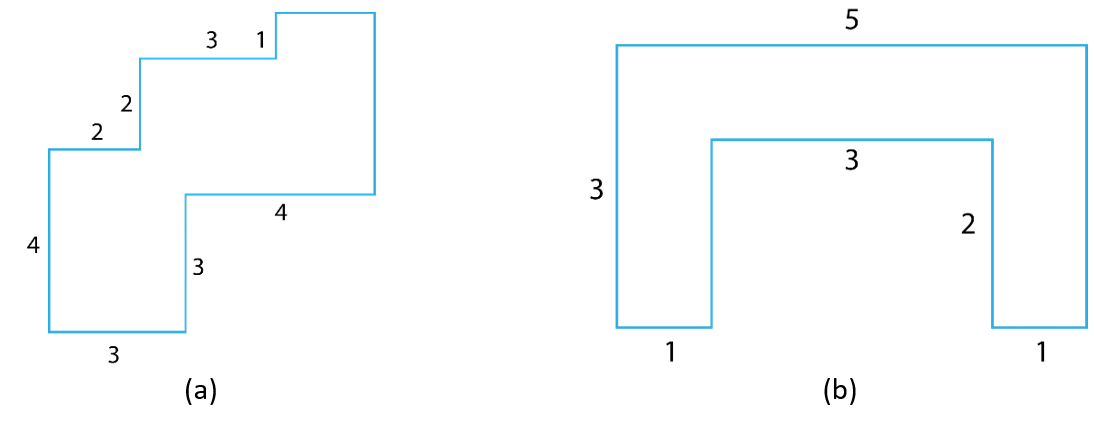Sol.10.

(a) Area = [(4 × 3) + (4 × 1) + (3 × 2) + (2 × 2) + (2 × 1)] sq cm
= 28 sq cm

(b) Area = [(3 × 1) + (3 × 1) + (3 × 1)] sq cm
= 9 sq cm

Ques. 11. Split the following shapes into rectangles and find their areas. (The measures are given in centimetres)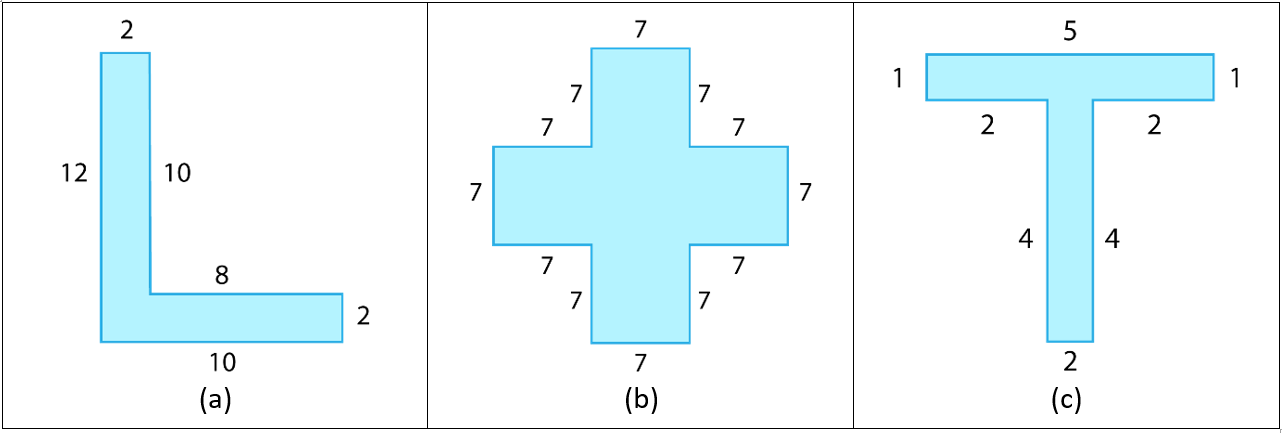Sol.11.

(a) Area = [(10 × 2) + (10 × 2)] sq cm
= 40 sq cm

(b) Area = [(7 × 7) × 5] sq cm
= 245 sq cm

(c) Area = [(5 × 1) + (4 × 1)] sq cm
= 9 sq cm

Ques. 12. How many tiles whose length and breadth are 12 cm and 5 cm respectively will be needed to fit in a rectangular region whose length and breadth are respectively:
(a) 100 cm and 144 cm

(b) 70 cm and 36 cm.

Sol.12. Area of a tile = 12 × 5 sq cm
= 60 sq cm

(a) Area of rectangular region = 100 × 144 sq cm
= 14,400 sq cm
No. of tiles required = 14400/60
= 240 tiles

(b) Area of rectangular region = 70 × 36 sq cm
= 2520 sq cm
No. of tiles required = 2520/60
= 42 tiles

With this we come to the end of NCERT Solutions for Class 6 Maths Chapter 10 Algebra. We hope these helped you study your subject.